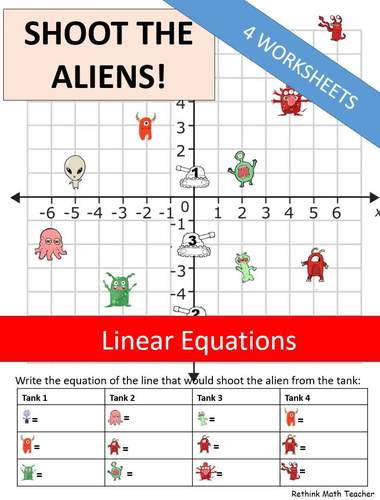# Linear Equations - Shoot the Aliens - 4 WorksheetsSubject
Resource Type
File Type

PDF

(472 KB|4 pages)
Product Rating
4.0
(1 Rating)
Standards
• Product Description
• StandardsNEW

A fun way to reinforce graphing linear equation in y = mx + b format. These 4 worksheets show aliens on a coordinate plane with tanks on the y-intercept. The students must shoot the alien by writing the correct formula from the tank to the alien.

Solve linear equations and inequalities in one variable, including equations with coefficients represented by letters.
Solve linear equations with rational number coefficients, including equations whose solutions require expanding expressions using the distributive property and collecting like terms.
Give examples of linear equations in one variable with one solution, infinitely many solutions, or no solutions. Show which of these possibilities is the case by successively transforming the given equation into simpler forms, until an equivalent equation of the form 𝘹 = 𝘢, 𝘢 = 𝘢, or 𝘢 = 𝘣 results (where 𝘢 and 𝘣 are different numbers).
Solve linear equations in one variable.
Total Pages
4 pages
Included
Teaching Duration
N/A
Report this Resource to TpT
Reported resources will be reviewed by our team. Report this resource to let us know if this resource violates TpT’s content guidelines.